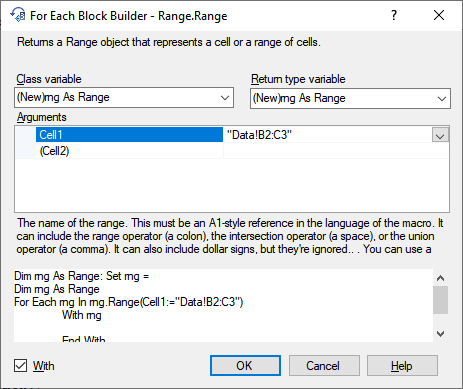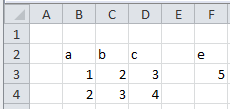﻿ VBA For Each cell in range

# Using For Each in Excel rangeAs the image above shows - taken from the Excel VBA menu-(download here...), there are many ways one can specify ranges to loop through. In this article several ways are discussed to do this.

## For Each cell in a rangeOne of the most common things you will do when programming VBA in Excel is looping though a collection of cells in a specified range, as in the example below which prints the Address and Value of 4 cells on the 'Data' worksheet to the immediate window: \$B\$2:a, \$C\$2:b, \$B\$3:1, \$C\$3:2.

``````Dim rng As Range: Set rng = Application.Range("Data!B2:C3")
Dim cel As Range
For Each cel In rng.Cells
With cel
Debug.Print .Address & ":" & .Value
End With
Next cel``````

### Loop through the cells in a rowThe code below shows how to loop through the cells in the row with `RowIndex:=2`. Applied to the data in the sheet on the right this will return 1, 2. From this we see that rows are counted from the starting point of rng, so the row is 3 on the worksheet, 2 inside rng. Also, only cells inside the set range rng are taken.

``````Dim rng As Range: Set rng = Application.Range("Data!B2:C3")
Dim i As Integer
For i = 1 To rng.Rows.Count
Debug.Print rng.Cells(RowIndex:=2, ColumnIndex:=i).Value
Next``````

### Loop through the cells in a column

The code below shows how to loop through the cells in the column with `ColumnIndex:=B`. Applied to the data in the sheet on the right this will return a, 1, 2. From this we see that columns are counted from the starting point of rng, so the column is C on the worksheet, B inside rng. Also, only cells inside the set range rng are taken.

``````Dim rng As Range: Set rng =
Dim i As Integer
For i = 1 To rng.Rows.Count
Debug.Print rng.Cells(RowIndex:=i, ColumnIndex:="B").Value
Next``````

### Loop through the columns in a range

The code below shows how to loop through the columns in the Range `B2:C4`. Applied to the data in the sheet on the right this will return 2, 3. From this we see that columns are counted from the starting point of the worksheet.

``````Dim rng As Range: Set rng = Application.Range("B2:C4")
Dim col As Range
For Each col In rng.Columns
Debug.Print col.Column
Next col``````

### Loop through the rows in a range

The code below shows how to loop through the rows in the Range `B2:C4`. Applied to the data in the sheet on the right this will return 2, 3, 4. From this we see that rows are counted from the starting point of the worksheet.

``````Dim rng As Range: Set rng = Application.Range("B2:C4")
Dim col As Range
For Each col In rng.Rows
Debug.Print col.Row
Next col``````

### Loop through the areas in a rangeOften we assume a range to have a rectangular shape, but this need not be the case. The example sheet on the right shows a selection containing two areas: `Selection.Address` returns \$B\$2:\$C\$3,\$F\$2:\$F\$3. Such a situation may also occur as a result of the `Intersect` method, or other causes. To handle the two ranges separately can can pick then from the Areas collection:

``````Dim rng As Range: Set rng = Application.Selection
Dim rngArea As Range
For Each rngArea In rng.Areas